## Set Predefined or Custom Formulas

You can set custom formulas using the Define Custom Formula icon.

The following illustration shows the Define Custom Formula icon.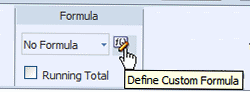Description of the illustration xdo11g_le_cust_form.gif

The Formula group of commands is available from the following tabs:

• Column tab

• Total Cell tab

• Chart Measure Field tab

• Pivot Table Data tab

Note that not all options are applicable to each component type.

The table provides definitions of predefined formulas.

The menu provides the predefined formulas that are described in the following table.

Formula Description

No Formula

Removes any mathematical formula from a numeric column.

Blank Text

Removes all data and inserts blank text.

Count

Returns the count of the number of occurrences of the element in the current group.

Count Distinct

Returns a count of the distinct values of an element in the current group.

Summation

Sums the values of the element in the current group.

Average

Displays the average of the values in the current group.

Maximum

Displays the highest value of all occurrences in the current group.

Minimum

Displays the lowest value of all occurrences in the current group.

For non-numeric data, only the following formula options are supported:

• Blank Text

• Count

• Count Distinct

### Apply a Custom Formula

Click Define Custom Formula to define your own formula for a component. The Function dialog enables you to define Basic Math, Context, and Statistical functions in the layout.

The following figure shows the Function dialog: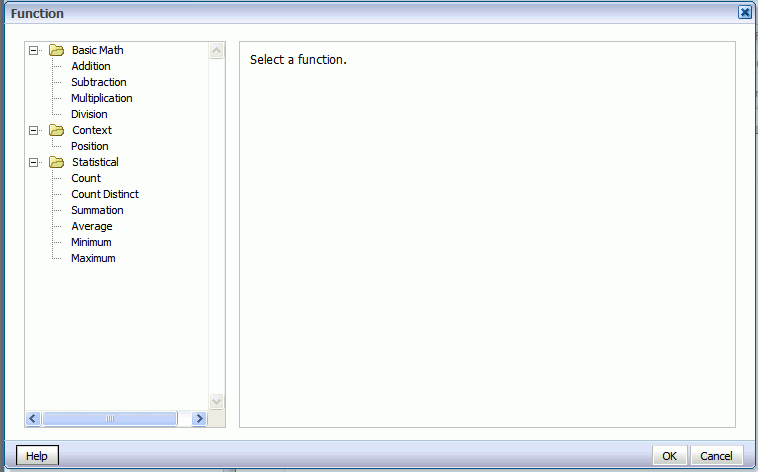Description of the illustration xdo11g_le_formula_new.gif

#### About the Basic Math Functions

When you click one of the basic math functions, you are prompted to define the appropriate parameters for the function. You can enter a constant value, select a field from the data, or create a nested function to supply the value.

In the Function dialog, clicking the Multiplication function displays prompts to enter the multiplicand and the multiplier. The example shows that the multiplicand is the value of the Amount Sold field. The multiplier is the constant value.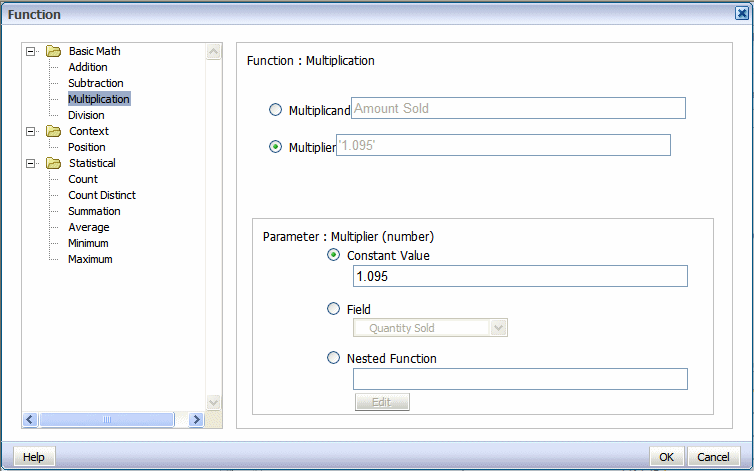Description of the illustration xdo11g_le_formula_new_mult.gif

#### About the Statistical Math Functions

When you click one of the statistical math functions you are prompted to define the appropriate parameter for the function. You can select a field from the data, or create a nested function to supply the values.

In the following figure, clicking the Average function displays prompts for you to specify the source of the values for which to calculate the average.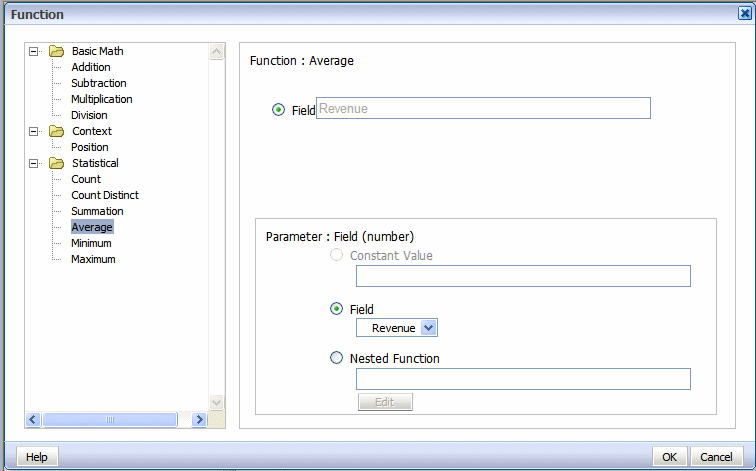Description of the illustration xdo11g_le_formula_stat.gif

### Apply a Custom Formula: Examples

Follow these examples to understand custom formula.

Example 3-1 Example 1: Subtraction

The following figure shows data for Revenue and Cost for each Office: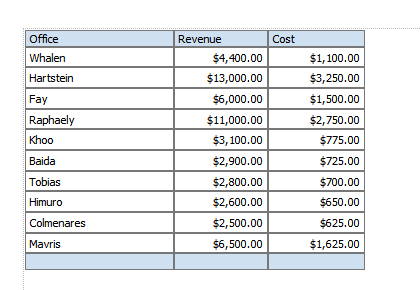Description of the illustration xdo11g_le_custform_exc.gif

Using a custom formula, you can add a column to this table to calculate Profit (Revenue - Cost).

1. Add another numeric data column to the table. For example, drag another instance of Revenue to the table, as shown in the following figure: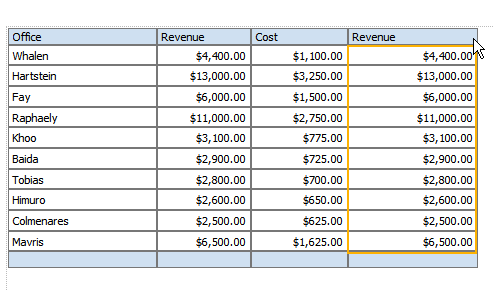Description of the illustration xdo11g_le_custform_exc1.gif
2. With the table column selected, click Define Custom Formula.

3. In the Function dialog select Subtraction from the list, as shown in the following figure. Because the source data for the column is Revenue, by default the Minuend and the Subtrahend both show the Revenue element.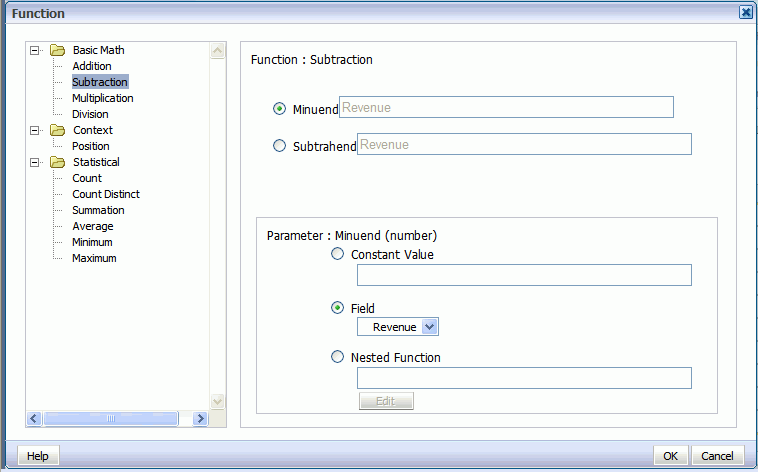Description of the illustration xdo11g_le_formula_ex_subt1.gif

4. Select Subtrahend, then in the Parameter region, select Field and choose the Cost element, as shown in the following figure: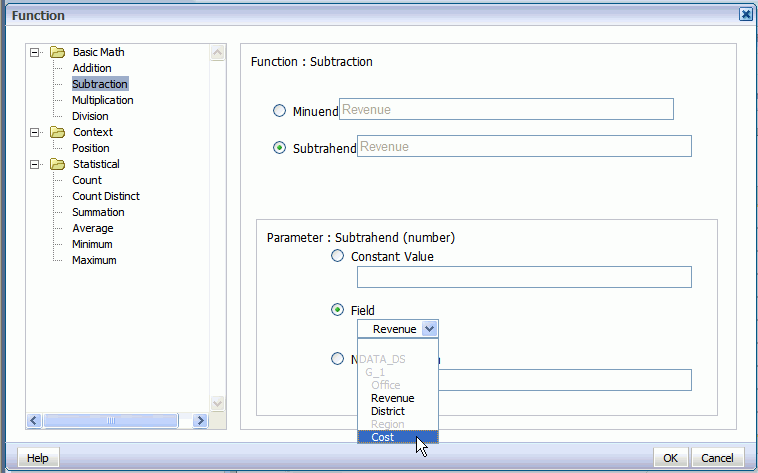Description of the illustration xdo11g_le_formula_ex_subt2.gif

The dialog is updated to show that the formula is now Revenue minus Cost, as shown in the following figure: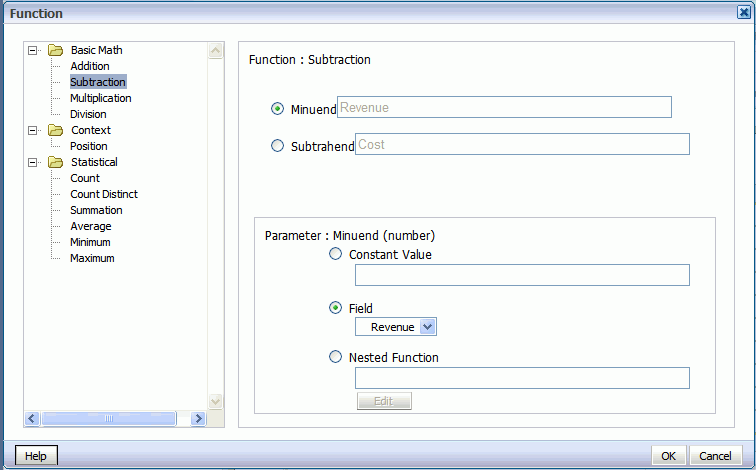Description of the illustration xdo11g_le_formula_ex_subt3.gif

5. Click OK to close the dialog.

6. The table column displays the custom formula. Edit the table column header title, and now the table has a Profit column, as shown in the following figure: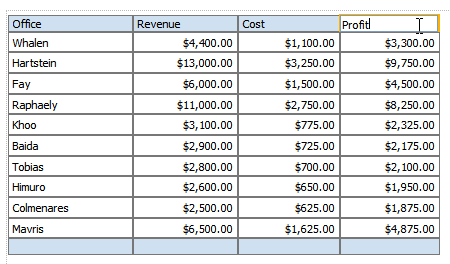Description of the illustration xdo11g_le_custform_exc6.gif

Example 3-2 Example 2: Nested Function

This example uses a nested function to create a column that shows Revenue less taxes.

1. Add another numeric data column to the table. For example, drag another instance of Revenue to the table, as shown in the following figure: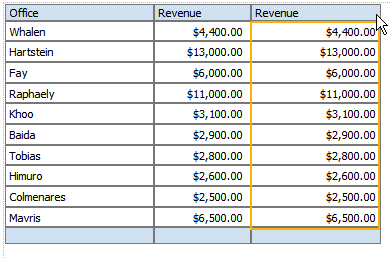Description of the illustration xdo11g_le_formula_nest1.gif
2. With the table column selected, click Define Custom Formula.

3. In the Function dialog select Subtraction from the list. Because the source data for the column is Revenue, by default the Minuend and the Subtrahend both show the Revenue element, as shown in the following figure:Description of the illustration xdo11g_le_formula_ex_subt1.gif

4. Select Subtrahend, then in the Parameter region, select Nested Function and click Edit, as shown in the following figure.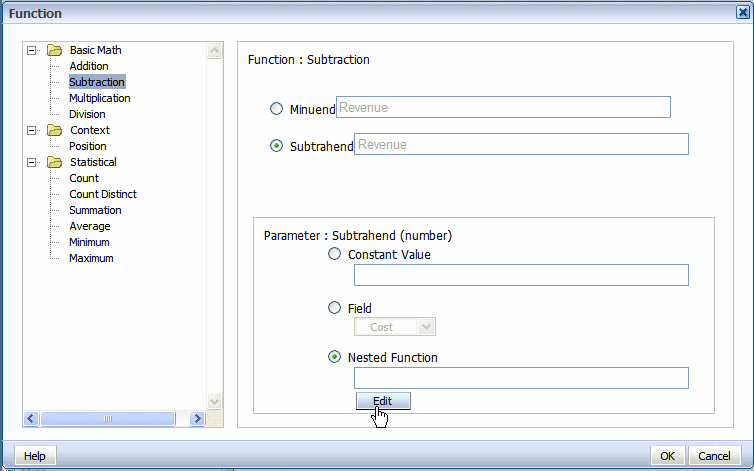Description of the illustration xdo11g_le_formula_ex2nes.gif

A second Function dialog is displayed to enable you to define the nested function. In this case the nested function is Revenue times a constant value (tax rate of .23), as shown in the following figure: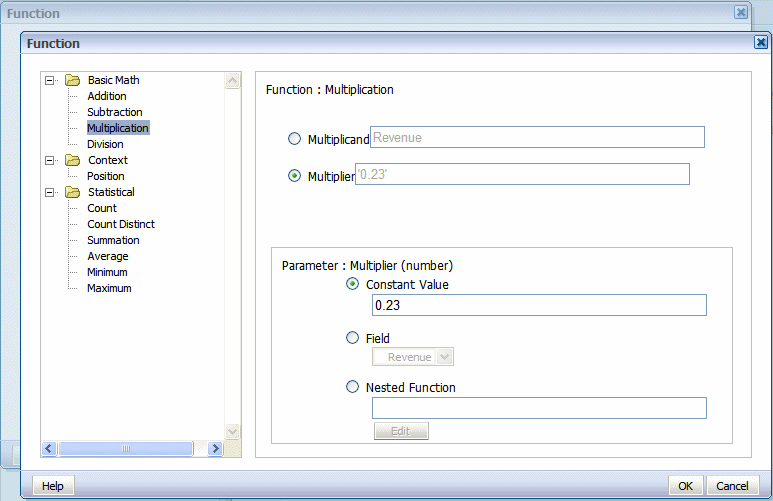Description of the illustration xdo11g_le_formula_exnes2.gif

5. Click OK to close the dialog. The primary Function dialog now shows the nested function as the source of the subtrahend, as shown in the following figure: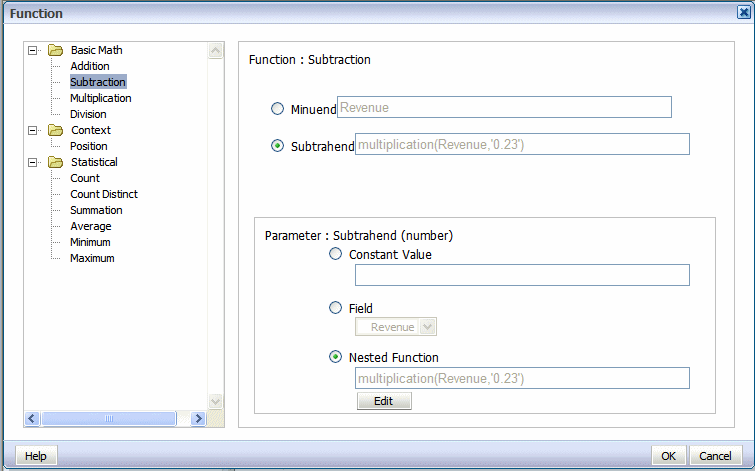Description of the illustration xdo11g_le_formula_exnes3.gif

6. Click OK to close the Function dialog. The table column displays the custom formula. Edit the table column header label, and now the table displays the custom function, as shown in the following figure: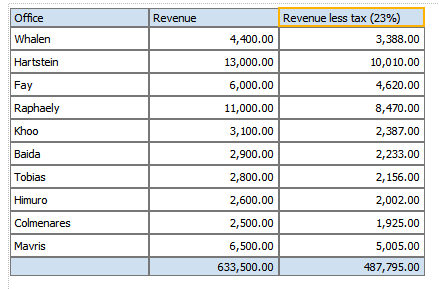Description of the illustration xdo11g_le_formula_exnes4.gif